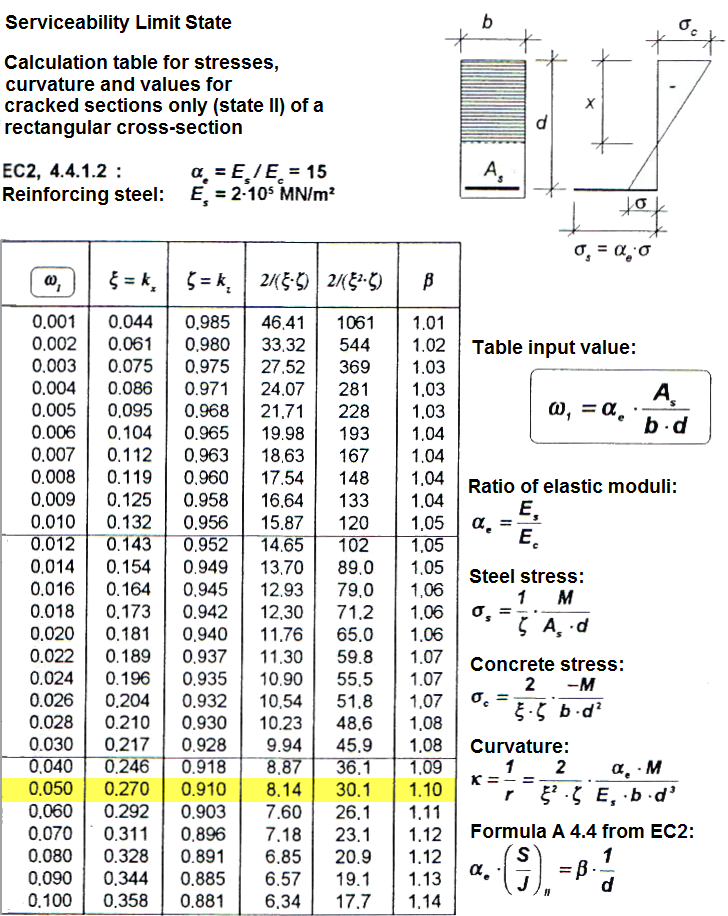# RF-CONCRETE Members – Online Manual Version 5

Online manuals, introductory examples, tutorials, and other documentation.

# 9.1.4 Curvature for Cracked Sections (State II)

### Curvature for Cracked Sections (State II)

Curvature due to loading

When characteristic loads are applied, concrete shows linear elastic behavior. The concrete stress distributed over the compression zone is assumed to be triangular.

The depth of the concrete compression area can be determined as follows:

The tension stress in the reinforcement is determined with MEd = 18.50 kNm as follows:

The curvature in the final crack state is determined as follows:

where

Curvature due to shrinkage

In manual calculations, the curvature for cracked sections (state II) is determined by means of a table from  (see Figure 9.2).

Total curvatureFigure 9.2 Calculation table for cracked sections only (state II) from 
Literatur
  Heydel, Günter, Krings, Wolfgang u. Hermann, Horst. Stahlbeton im Hochbau nach EC2: Einführung und Anwendungsbeispiele. Ernst & Sohn Verlag, 1995